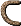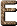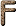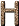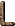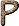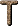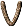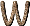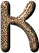K Spectral type for orange stars, such as Arcturus, Aldebaran, and Alpha Centauri B. K type stars are somewhat cooler than the Sun. [C95] K A quantum number which refers to the component of angular momentum around a molecule's axis of symmetry. [H76] KA spectral line in the X-ray region (= 0.334 Å), produced by the transition between the lowest level of the K shell and the lowest level of the L shell. [H76] K capture Capture by a nucleus of one of the electrons in its innermost shell, accompanied by the emission of X-rays. [H76] K component The inner part of the Solar corona (the gaseous phase) which emits a continuous spectrum without absorption lines. Physically, the K component results from Thomson scattering of photospheric radiation by free electrons in the corona. The K component is polarized and decreases rapidly with distance from the Sun. (from the German Kontinuum) [H76] K corona See K component. [H76] K correction In extragalactic studies, the observed luminosities and colors of objects participating in the expansion of the Universe are known to require procedural corrections for two physically distinct, but redshift-related, reasons: (1) the stretching of the restframe spectral energy distribution across the observer's (fixed) bandpass, and (2) the redshift/displacement of the restframe wavelength across and into the observer's filter. The first effect is purely a function of the redshift, z, where the effective filter width decreases in proportion to 1 / (1 + z). The second effect is primarily a function of the spectral energy distribution of the source, rising and falling with the relative continuum (or emission-line) strength being sampled for that filter and redshift combination. Failure to appreciate these "technical effects" associated with the redshift led to a very early claim of rapid evolution of nearby elliptical galaxies, the so-called Stebbins-Whitford Effect. (also called K term) [BFM99] K edge The absorption edge of the K shell (see absorption edges). [H76] K electron An electron in the K shell. [H76] k line A Mg II resonance line at 2795.5 Å. [H76] K line A spectral line of singly ionized calcium at 3933 Å (see Fraunhofer lines). [H76] K magnitude The magnitude derived from observations at 2.2 microns. [H76] K meson or kaon The name of particular spin-0 mesons with non-zero strangeness quantum numbers. [CD99] K shell The innermost shell, or energy level, of an atom. All elements heavier than hydrogen have a filled K shell, which consists of two 1s electrons orbiting the nucleus. [H76] K star Stars of spectral type K are cool, orange to red stars with surface temperatures of about 3600-5000 K. Their spectra resemble those of sunspots, in which the Hydrogen lines have been greatly weakened. The H and K lines (q.v.) reach their greatest intensity. Strongest lines are Ca I (4227 Å) and the G-band (4303 Å). Examples are Arcturus and Aldebaran. [H76] Kaluza-Klein theory (a) Class of theories incorporating extra curled-up dimensions, together with quantum mechanics. [G99] (b) An early attempt to unify general relativity and electromagnetism by working in five dimensions. The electromagnetic field was obtained by curling up or compactifying the extra dimension. With the advent of higher dimensional theories such as superstrings, the Kaluza-Klein approach came back into fashion. [P88] KAO Kuiper Airborne Observatory. [LLM96] kaon (K-meson) variety of strange meson. [D89] kaon (K-meson) A type of meson. There are four types of meson: positively charged, negatively charged, and two types of neutral particle. They are strongly interacting bosons of zero spin with several alternative modes of decay. [DC99] Kapteyn's Star (a) A nearby star discovered in 1897 by Jacobus Kapteyn. It is a red subdwarf that lies 13 light-years away and is both the nearest halo star to the Sun and the nearest star that orbits the Galaxy backward. (HD 33793, CD -45°1841) [C95] (b) A high-velocity (radial velocity +242 km s-1) M0 subdwarf 3.9 pc distant. [H76] Kapteyn Universe An incorrect model for the Galaxy proposed by Jacobus Kapteyn in which the Milky Way was small and the Sun located at or near the Galaxy's center. [C95] kayser A wavenumber. (= cm-1) [H76] Keller-Meyerott opacity Opacity taken from the tables derived by G. Keller and R. Meyerott for assumed mixtures of hydrogen, helium, and heavy elements ranging from X = 99%, Y = 1%, Z = 0 to X = 0, Y = 50%, Z = 50%. [H76] kelvin (a) The temperature scale that astronomers usually use. On the Kelvin scale, the coldest possible temperature is 0 degrees. This corresponds to -273° Celsius or -460° Fahrenheit. Room temperature is about 295 degrees Kelvin. [C95] (b) The SI unit of thermodynamic temperature. "The kelvin, unit of thermodynamic temperature, is the fraction 1/273.16 of the thermodynamic temperature of the triple point of water". [13th CGPM (1967), Resolution 4]. [H76] kelvin (K) The kelvin is the fundamental unit of temperature in the SI system. It is defined as the fraction 1/273.16 of the thermodynamic temperature of the triple point of water. The definition was adopted by the 16th CGPM (1968) when it was decided to change the name of the unit from the degree kelvin (°K) to the kelvin (K). It is named after Lord Kelvin (1824-1907), one of the outstanding physicists of the nineteenth century. [KM92] Kelvin contraction The contraction of a star contemplated by Kelvin and Helmholtz as a consequence of a star's radiating its thermal energy. It is currently believed that the contraction of a star occurs in this manner in its pre-main-sequence evolution. (also called Kelvin-Helmholtz contraction) [H76] Kelvin scale A temperature scale with the same divisions as the Celsius (centigrade) scale and with the zero point at 0° absolute. (Room temperature is about 295 K). [H76] Kelvin scale the `natural' or `absolute' scale of temperature, on which the value of temperature corresponds roughly to the typical thermal energy. The Kelvin temperature is approximately the Celsius temperature plus 273 degrees. [D89] Kelvin timescale The time it takes a star to contract gravitationally from infinite radius down to its present radius by radiating its thermal energy (for the Sun, about 2-3 × 107 years). The Kelvin time is roughly equal to (gravitational binding energy) / luminosity. (Compare nuclear time scale). [H76] Kerr black hole A rotating, axisymmetric black hole based on Kerr's 1963 solution to Einstein's field equations. [H76] Kennelly-Heaviside layer Former name for the D and E layers (q.v.). [H76] Kepler's Laws (1) Each planetary orbit is an ellipse with the Sun at one focus. (2) (law of areas) Equal areas are swept out in equal times. (3) (harmonic law) The square of the period is proportional to the cube of the distance. Newton's generalized formula for the third law is P2 = 42 a3 / [G (m1 + m2)]. [H76] Keplerian orbit The orbit of a spherical particle of a finite mass around another spherical particle, also of finite mass, by virtue of the gravitational attraction between them. In the Bohr-Sommerfeld picture of atoms, the electrons are considered as describing Keplerian orbits in the field of the positive nucleus by virtue of the inverse square electric attraction between the electrons and the nuclei. [H76] Keplerian rotation curve A rotation curve is a plot of speed versus distance from the center of an astronomical system. If most of the mass of the system is concentrated at the center, as in a solar system, then the speed of any orbiting body, such as a planet, is inversely proportional to the square root of its distance from the center. This last situation produces a Keplerian rotation curve. (See rotation curve.) [LB90] Keplerian telescope (astronomical telescope) The most common type of refracting telescope arrangement, consisting of converging objective and eyepiece. Unlike the Galilean telescope, it provides an inverted image and has a greater length. However the field of view is larger and image quality is higher. The angular magnification is given by (fO + fE); the lens separation is (fO + fE). For terrestrial use an inverting lens can be included between the objective and the eyepiece. This is placed so that it is a distance 2fI (fI is its focal distance) from the first image formed by the objective. An erect image is formed a distance 2f behind the inverting lens, arranged to be at the principal focus of the eyepiece. The magnification is not affected but the distance from objective to eyepiece is now fO + fE + 4f. [DC99] Kepler's Supernova A Type I supernova (SN Oph 1604) whose light reached Earth in 1604. If H0 = 50, then Kepler's supernova is out in the galactic halo at a distance of 12.1 kpc and 1.4 kpc above the galactic plane, according to van den Bergh. Kepler's supernova is the prototype of Type I supernovae; at its brightest it reached an apparent magnitude of about -2.2. (3C 358) [H76] kernel The set of points mapped into zero. [H76] Kerr solution A solution to Einstein's equations describing the gravity produced by a spinning point of mass. [LB90] KeV one thousand electron volts. [D89] Keyhole Nebula An old name for Eta Carinae. [H76] Killing vector When it exists, the Killing vector describes symmetry properties of spacetime. If spacetime admits a timelike Killing vector, then it is stationary; similarly, axial symmetry is derived from a spacelike Killing vector. [H76] kilo- A prefix meaning 103. [H76] kilogram The SI basic unit of mass (not of weight or of force). kilogram is equal to the mass of 1.000028 cubic decimeters of water at the temperature of its maximum density. [H76] kilogram (kg) The fundamental unit of mass in the metric system. It was originally supposed to represent the mass of a cubic decimetre of water at the temperature of its maximum density. Subsequent work showed that the cube used for the determination of the kilogram of 1799, known as the Kilogramme des Archives, had a volume of 1.000028 cubic decimetres, thereby making it too heavy. At the Convention du Mètre held in Paris in May 1875 it was decided to make a new international kilogram which would be an exact copy of the Kilogramme des Archives. The new standard, known as the International Prototype Kilogramme, was established in 1889 and copies of it were sent to the various bodies responsible for weights and measures throughout the world. Copy No. 18 was sent to England, No. 20 to the USA. The British copy is kept at the National Physical Laboratory. The standard itself is a platinum iridium cylinder preserved at the International Bureau of Weights and Measures at Sèvres, near Paris, and is unique in that it is now the only one of the seven basic SI units which is man-made. The vault in which it is housed is secured by three locks. A separate key is held by the director at Sèvres, the chairman for weights and measures and the archivist of France. However it seems probable that, early in the twenty first century, the mass of the international prototype kilogram will be redefined either in terms of the mass of a silicone crystal or by an appropriate combination of the values of some readily measurable and defined physical constants such as the fine structure constant Rydberg constant etc. In the British Parliament during the 1962-1963 Session a weights and measures Bill was considered in which the Imperial pound was defined in the following terms: 'the pound shall be 0.45359237 kilograms exactly'. This Act, passed in 1963, makes the kilogram the fundamental unit of mass in both the metric and the foot pound second systems of units. [JM92] kilometer per second The unit of speed in astronomy. One kilometer per second is 2237 miles per hour - five times the speed of an airplane. [C95] kiloparsec A measure of distance equal to 1,000 parsecs, or about 3,000 light years. [LB90] kinematic relativity Theory proposed by Edward Milne as a viable alternative to Einstein's general theory of relativity, and based generally on kinematics (the science of pure motion, without reference to matter or force), from which Milne successfully derived new systems of dynamics and electrodynamics. [A84] kinematics The branch of mechanics that studies bodies undergoing continuous change of position. Whereas dynamics takes into account mass, force, distance, and time, kinematics is concerned only with distance and time. [H76] kinetic energy The energy associated with motion; the work that must be done to change a body from a state of rest to a state of motion, equal to 1/2 mv2 for a body of mass m moving at velocity v. [Silk90] kinetic temperature A measure of the average random motion of the particles in a system. [H76] Kirchhoff's laws (1) To each chemical species there corresponds a characteristic spectrum. (2) Every element is capable of absorbing the radiation which it is able to emit; this is the phenomenon of the reversal of the lines. [H76] Kirkwood gaps Regions in the asteroid zone which have been swept clear of asteroids by the perturbing effects of Jupiter. They were named for the American astronomer Daniel Kirkwood. [H76] KL nebula See Kleinmann-Low nebula. [H76] Klein-Alfvén cosmology A cosmology in which the observed expansion of the Universe results from the bounce of an originally collapsing cloud of matter and antimatter. The bounce is caused by radiation pressure generated by annihilations when the cloud reaches high density (10-2 cm-3). [H76] Klein-Gordon equation A fundamental equation of relativistic quantum field theory. [G99] Klein-Nishina formula An expression for the total or differential cross section for the Compton scattering of a photon by a free electron according to Dirac's electron theory. [H76] Kleinmann-Low nebula A cool (< 600 K) extended infrared source in the Orion Nebula, about 1' NW of the Trapezium and about 12" south of the BN nebula, discovered in 1967. It dominates the infrared emission at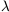20 µ, and a CO cloud is centered on it. It is probably a collapsing cloud of 102-103 M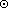in which protostars are embedded. (KL nebula) [H76] klystron A type of electron tube used in radar and high-frequency radio work. [H76] knock-on spectrum A spectrum of particles that are being built up - the inverse of a spallation spectrum. [H76] Knudsen number The ratio of the mean free path length of the molecules in a fluid to a characteristic length of the structure in the fluid stream. [H76] Kolmogorov-Smirnov test A nonparametric test used in statistics. The Kolmogorov statistic is simply the magnitude of the maximum deviation between the integral distribution function of a sample and the theoretical distribution one wishes to test. [H76] Kramers's opacity The opacity of stellar material derived by Kramers, who in 1923 carried out theoretical calculations of stellar opacity as a function of chemical composition. [H76] KREEP Lunar basaltic material rich in radioactive elements. (from K for potassium, REE for rare-earth elements, P for phosphorus) [H76] Krüger 60 AB A faint, twelfth-magnitude dM binary (P = 44.5 years) in the Solar neighborhood (3.93 pc distant). It may be a subluminous star. [H76] Kruskal diagram A spacetime diagram exhibiting the properties of the Schwarzschild metric by eliminating the formal singularity that appears at the Schwarzschild radius in the form in which the metric is usually written. It exhibits the character of the horizon that appears at the Schwarzschild radius and illustrates the true nature of the singularity at the center. The Kruskal diagram fully describes spacetime in the vicinity of a black hole all the way down to the singularity. [H76] Krypton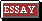A colorless odorless monatomic element of the rare-gas group, known to form unstable compounds with fluorine. It occurs in minute quantities (0.001% by volume) in air. Krypton is used in fluorescent lights. Symbol: Kr; m.p. -156.55°C; b.p. -152.3°C; d. 3.749 (0°C) kg m-3; p.n. 36; r.a.m. 83.80. [DC99] Krzeminski's star See Cen X-3. [H76] Kuiper bands Bands in the spectra of Uranus and Neptune at wavelengths of 7,500 Å, indicating the presence of methane. [A84] kurtosis The peakedness or flatness in the graphical representation of a statistical distribution. [H76]